# 6.4 Arc length of a curve and surface area  (Page 5/8)

 Page 5 / 8

## Calculating the surface area of a surface of revolution 2

Let $f\left(x\right)=y=\sqrt{3x}.$ Consider the portion of the curve where $0\le y\le 2.$ Find the surface area of the surface generated by revolving the graph of $f\left(x\right)$ around the $y\text{-axis}.$

Notice that we are revolving the curve around the $y\text{-axis},$ and the interval is in terms of $y,$ so we want to rewrite the function as a function of y . We get $x=g\left(y\right)=\left(1\text{/}3\right){y}^{3}.$ The graph of $g\left(y\right)$ and the surface of rotation are shown in the following figure.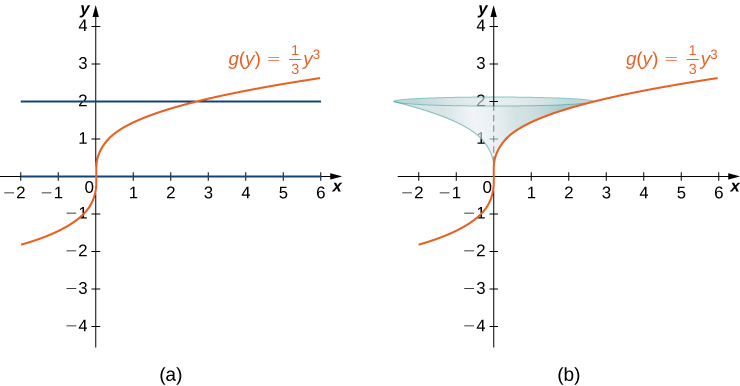(a) The graph of g ( y ) . (b) The surface of revolution.

We have $g\left(y\right)=\left(1\text{/}3\right){y}^{3},$ so ${g}^{\prime }\left(y\right)={y}^{2}$ and ${\left({g}^{\prime }\left(y\right)\right)}^{2}={y}^{4}.$ Then

$\begin{array}{cc}\hfill \text{Surface Area}& ={\int }_{c}^{d}\left(2\pi g\left(y\right)\sqrt{1+{\left({g}^{\prime }\left(y\right)\right)}^{2}}\right)dy\hfill \\ & ={\int }_{0}^{2}\left(2\pi \left(\frac{1}{3}{y}^{3}\right)\sqrt{1+{y}^{4}}\right)dy\hfill \\ & =\frac{2\pi }{3}{\int }_{0}^{2}\left({y}^{3}\sqrt{1+{y}^{4}}\right)dy.\hfill \end{array}$

Let $u={y}^{4}+1.$ Then $du=4{y}^{3}dy.$ When $y=0,$ $u=1,$ and when $y=2,$ $u=17.$ Then

$\begin{array}{cc}\hfill \frac{2\pi }{3}{\int }_{0}^{2}\left({y}^{3}\sqrt{1+{y}^{4}}\right)dy& =\frac{2\pi }{3}{\int }_{1}^{17}\frac{1}{4}\sqrt{u}du\hfill \\ & =\frac{\pi }{6}{\left[\frac{2}{3}{u}^{3\text{/}2}\right]\phantom{\rule{0.2em}{0ex}}|}_{1}^{17}=\frac{\pi }{9}\left[{\left(17\right)}^{3\text{/}2}-1\right]\approx 24.118.\hfill \end{array}$

Let $g\left(y\right)=\sqrt{9-{y}^{2}}$ over the interval $y\in \left[0,2\right].$ Find the surface area of the surface generated by revolving the graph of $g\left(y\right)$ around the $y\text{-axis}.$

$12\pi$

## Key concepts

• The arc length of a curve can be calculated using a definite integral.
• The arc length is first approximated using line segments, which generates a Riemann sum. Taking a limit then gives us the definite integral formula. The same process can be applied to functions of $y.$
• The concepts used to calculate the arc length can be generalized to find the surface area of a surface of revolution.
• The integrals generated by both the arc length and surface area formulas are often difficult to evaluate. It may be necessary to use a computer or calculator to approximate the values of the integrals.

## Key equations

• Arc Length of a Function of x
$\text{Arc Length}={\int }_{a}^{b}\sqrt{1+{\left[{f}^{\prime }\left(x\right)\right]}^{2}}\phantom{\rule{0.2em}{0ex}}dx$
• Arc Length of a Function of y
$\text{Arc Length}={\int }_{c}^{d}\sqrt{1+{\left[{g}^{\prime }\left(y\right)\right]}^{2}}\phantom{\rule{0.2em}{0ex}}dy$
• Surface Area of a Function of x
$\text{Surface Area}={\int }_{a}^{b}\left(2\pi f\left(x\right)\sqrt{1+{\left({f}^{\prime }\left(x\right)\right)}^{2}}\right)dx$

For the following exercises, find the length of the functions over the given interval.

$y=5x\phantom{\rule{0.2em}{0ex}}\text{from}\phantom{\rule{0.2em}{0ex}}x=0\phantom{\rule{0.2em}{0ex}}\text{to}\phantom{\rule{0.2em}{0ex}}x=2$

$2\sqrt{26}$

$y=-\frac{1}{2}x+25\phantom{\rule{0.2em}{0ex}}\text{from}\phantom{\rule{0.2em}{0ex}}x=1\phantom{\rule{0.2em}{0ex}}\text{to}\phantom{\rule{0.2em}{0ex}}x=4$

$x=4y\phantom{\rule{0.2em}{0ex}}\text{from}\phantom{\rule{0.2em}{0ex}}y=-1\phantom{\rule{0.2em}{0ex}}\text{to}\phantom{\rule{0.2em}{0ex}}y=1$

$2\sqrt{17}$

Pick an arbitrary linear function $x=g\left(y\right)$ over any interval of your choice $\left({y}_{1},{y}_{2}\right).$ Determine the length of the function and then prove the length is correct by using geometry.

Find the surface area of the volume generated when the curve $y=\sqrt{x}$ revolves around the $x\text{-axis}$ from $\left(1,1\right)$ to $\left(4,2\right),$ as seen here.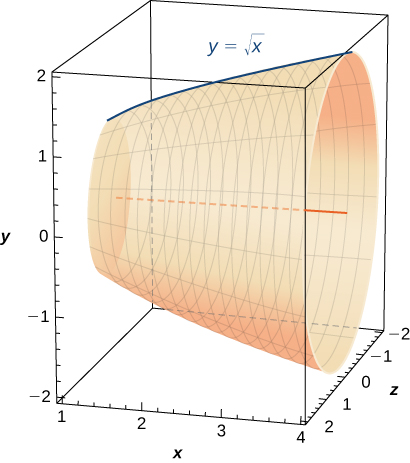$\frac{\pi }{6}\left(17\sqrt{17}-5\sqrt{5}\right)$

Find the surface area of the volume generated when the curve $y={x}^{2}$ revolves around the $y\text{-axis}$ from $\left(1,\phantom{\rule{0.2em}{0ex}}1\right)$ to $\left(3,9\right).$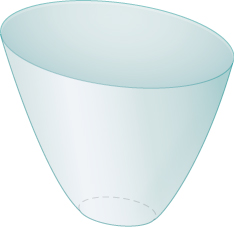For the following exercises, find the lengths of the functions of $x$ over the given interval. If you cannot evaluate the integral exactly, use technology to approximate it.

$y={x}^{3\text{/}2}$ from $\left(0,0\right)\phantom{\rule{0.2em}{0ex}}\text{to}\phantom{\rule{0.2em}{0ex}}\left(1,1\right)$

$\frac{13\sqrt{13}-8}{27}$

$y={x}^{2\text{/}3}$ from $\left(1,1\right)\phantom{\rule{0.2em}{0ex}}\text{to}\phantom{\rule{0.2em}{0ex}}\left(8,4\right)$

$y=\frac{1}{3}{\left({x}^{2}+2\right)}^{3\text{/}2}$ from $x=0\phantom{\rule{0.2em}{0ex}}\text{to}\phantom{\rule{0.2em}{0ex}}x=1$

$\frac{4}{3}$

$y=\frac{1}{3}{\left({x}^{2}-2\right)}^{3\text{/}2}$ from $x=2$ to $x=4$

[T] $y={e}^{x}$ on $x=0$ to $x=1$

$2.0035$

$y=\frac{{x}^{3}}{3}+\frac{1}{4x}$ from $x=1\phantom{\rule{0.2em}{0ex}}\text{to}\phantom{\rule{0.2em}{0ex}}x=3$

$y=\frac{{x}^{4}}{4}+\frac{1}{8{x}^{2}}$ from $x=1\phantom{\rule{0.2em}{0ex}}\text{to}\phantom{\rule{0.2em}{0ex}}x=2$

$\frac{123}{32}$

$y=\frac{2{x}^{3\text{/}2}}{3}-\frac{{x}^{1\text{/}2}}{2}$ from $x=1\phantom{\rule{0.2em}{0ex}}\text{to}\phantom{\rule{0.2em}{0ex}}x=4$

$y=\frac{1}{27}{\left(9{x}^{2}+6\right)}^{3\text{/}2}$ from $x=0\phantom{\rule{0.2em}{0ex}}\text{to}\phantom{\rule{0.2em}{0ex}}x=2$

$10$

[T] $y=\text{sin}\phantom{\rule{0.2em}{0ex}}x$ on $x=0\phantom{\rule{0.2em}{0ex}}\text{to}\phantom{\rule{0.2em}{0ex}}x=\pi$

For the following exercises, find the lengths of the functions of $y$ over the given interval. If you cannot evaluate the integral exactly, use technology to approximate it.

$y=\frac{5-3x}{4}$ from $y=0$ to $y=4$

$\frac{20}{3}$

$x=\frac{1}{2}\left({e}^{y}+{e}^{\text{−}y}\right)$ from $y=-1\phantom{\rule{0.2em}{0ex}}\text{to}\phantom{\rule{0.2em}{0ex}}y=1$

$x=5{y}^{3\text{/}2}$ from $y=0$ to $y=1$

$\frac{1}{675}\left(229\sqrt{229}-8\right)$

[T] $x={y}^{2}$ from $y=0$ to $y=1$

$x=\sqrt{y}$ from $y=0\phantom{\rule{0.2em}{0ex}}\text{to}\phantom{\rule{0.2em}{0ex}}y=1$

$\frac{1}{8}\left(4\sqrt{5}+\text{ln}\left(9+4\sqrt{5}\right)\right)$

$x=\frac{2}{3}{\left({y}^{2}+1\right)}^{3\text{/}2}$ from $y=1$ to $y=3$

[T] $x=\text{tan}\phantom{\rule{0.2em}{0ex}}y$ from $y=0$ to $y=\frac{3}{4}$

$1.201$

[T] $x={\text{cos}}^{2}y$ from $y=-\frac{\pi }{2}$ to $y=\frac{\pi }{2}$

[T] $x={4}^{y}$ from $y=0\phantom{\rule{0.2em}{0ex}}\text{to}\phantom{\rule{0.2em}{0ex}}y=2$

$15.2341$

[T] $x=\text{ln}\left(y\right)$ on $y=\frac{1}{e}$ to $y=e$

For the following exercises, find the surface area of the volume generated when the following curves revolve around the $x\text{-axis}.$ If you cannot evaluate the integral exactly, use your calculator to approximate it.

$y=\sqrt{x}$ from $x=2$ to $x=6$

$\frac{49\pi }{3}$

$y={x}^{3}$ from $x=0$ to $x=1$

$y=7x$ from $x=-1\phantom{\rule{0.2em}{0ex}}\text{to}\phantom{\rule{0.2em}{0ex}}x=1$

$70\pi \sqrt{2}$

[T] $y=\frac{1}{{x}^{2}}$ from $x=1\phantom{\rule{0.2em}{0ex}}\text{to}\phantom{\rule{0.2em}{0ex}}x=3$

$y=\sqrt{4-{x}^{2}}$ from $x=0\phantom{\rule{0.2em}{0ex}}\text{to}\phantom{\rule{0.2em}{0ex}}x=2$

$8\pi$

$y=\sqrt{4-{x}^{2}}$ from $x=-1\phantom{\rule{0.2em}{0ex}}\text{to}\phantom{\rule{0.2em}{0ex}}x=1$

$y=5x$ from $x=1\phantom{\rule{0.2em}{0ex}}\text{to}\phantom{\rule{0.2em}{0ex}}x=5$

$120\pi \sqrt{26}$

[T] $y=\text{tan}\phantom{\rule{0.2em}{0ex}}x$ from $x=-\frac{\pi }{4}\phantom{\rule{0.2em}{0ex}}\text{to}\phantom{\rule{0.2em}{0ex}}x=\frac{\pi }{4}$

For the following exercises, find the surface area of the volume generated when the following curves revolve around the $y\text{-axis}\text{.}$ If you cannot evaluate the integral exactly, use your calculator to approximate it.

$y={x}^{2}$ from $x=0\phantom{\rule{0.2em}{0ex}}\text{to}\phantom{\rule{0.2em}{0ex}}x=2$

$\frac{\pi }{6}\left(17\sqrt{17}-1\right)$

$y=\frac{1}{2}{x}^{2}+\frac{1}{2}$ from $x=0\phantom{\rule{0.2em}{0ex}}\text{to}\phantom{\rule{0.2em}{0ex}}x=1$

$y=x+1$ from $x=0\phantom{\rule{0.2em}{0ex}}\text{to}\phantom{\rule{0.2em}{0ex}}x=3$

$9\sqrt{2}\pi$

[T] $y=\frac{1}{x}$ from $x=\frac{1}{2}$ to $x=1$

$y=\sqrt{x}$ from $x=1\phantom{\rule{0.2em}{0ex}}\text{to}\phantom{\rule{0.2em}{0ex}}x=27$

$\frac{10\sqrt{10}\pi }{27}\left(73\sqrt{73}-1\right)$

[T] $y=3{x}^{4}$ from $x=0$ to $x=1$

[T] $y=\frac{1}{\sqrt{x}}$ from $x=1$ to $x=3$

$25.645$

[T] $y=\text{cos}\phantom{\rule{0.2em}{0ex}}x$ from $x=0$ to $x=\frac{\pi }{2}$

The base of a lamp is constructed by revolving a quarter circle $y=\sqrt{2x-{x}^{2}}$ around the $y\text{-axis}$ from $x=1$ to $x=2,$ as seen here. Create an integral for the surface area of this curve and compute it.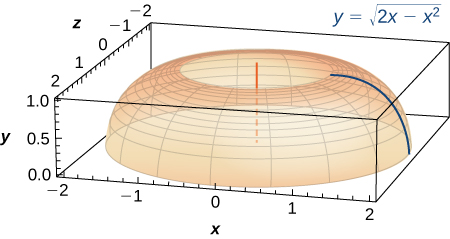$2\pi$

A light bulb is a sphere with radius $1\text{/}2$ in. with the bottom sliced off to fit exactly onto a cylinder of radius $1\text{/}4$ in. and length $1\text{/}3$ in., as seen here. The sphere is cut off at the bottom to fit exactly onto the cylinder, so the radius of the cut is $1\text{/}4$ in. Find the surface area (not including the top or bottom of the cylinder).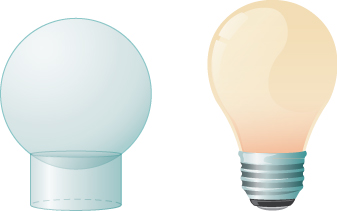[T] A lampshade is constructed by rotating $y=1\text{/}x$ around the $x\text{-axis}$ from $y=1$ to $y=2,$ as seen here. Determine how much material you would need to construct this lampshade—that is, the surface area—accurate to four decimal places.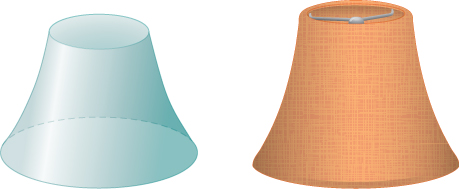$10.5017$

[T] An anchor drags behind a boat according to the function $y=24{e}^{\text{−}x\text{/}2}-24,$ where $y$ represents the depth beneath the boat and $x$ is the horizontal distance of the anchor from the back of the boat. If the anchor is $23$ ft below the boat, how much rope do you have to pull to reach the anchor? Round your answer to three decimal places.

[T] You are building a bridge that will span $10$ ft. You intend to add decorative rope in the shape of $y=5|\text{sin}\left(\left(x\pi \right)\text{/}5\right)|,$ where $x$ is the distance in feet from one end of the bridge. Find out how much rope you need to buy, rounded to the nearest foot.

$23$ ft

For the following exercises, find the exact arc length for the following problems over the given interval.

$y=\text{ln}\left(\text{sin}\phantom{\rule{0.2em}{0ex}}x\right)$ from $x=\pi \text{/}4$ to $x=\left(3\pi \right)\text{/}4.$ ( Hint: Recall trigonometric identities.)

Draw graphs of $y={x}^{2},$ $y={x}^{6},$ and $y={x}^{10}.$ For $y={x}^{n},$ as $n$ increases, formulate a prediction on the arc length from $\left(0,0\right)$ to $\left(1,1\right).$ Now, compute the lengths of these three functions and determine whether your prediction is correct.

$2$

Compare the lengths of the parabola $x={y}^{2}$ and the line $x=by$ from $\left(0,0\right)\phantom{\rule{0.2em}{0ex}}\text{to}\phantom{\rule{0.2em}{0ex}}\left({b}^{2},b\right)$ as $b$ increases. What do you notice?

Solve for the length of $x={y}^{2}$ from $\left(0,0\right)\phantom{\rule{0.2em}{0ex}}\text{to}\phantom{\rule{0.2em}{0ex}}\left(1,1\right).$ Show that $x=\left(1\text{/}2\right){y}^{2}$ from $\left(0,0\right)$ to $\left(2,\phantom{\rule{0.2em}{0ex}}2\right)$ is twice as long. Graph both functions and explain why this is so.

[T] Which is longer between $\left(1,\phantom{\rule{0.2em}{0ex}}1\right)$ and $\left(2,1\text{/}2\right)\text{:}$ the hyperbola $y=1\text{/}x$ or the graph of $x+2y=3?$

Explain why the surface area is infinite when $y=1\text{/}x$ is rotated around the $x\text{-axis}$ for $1\le x<\infty ,$ but the volume is finite.

determine the area of the region enclosed by x²+y=1,2x-y+4=0
How to use it to slove fraction
Hello please can someone tell me the meaning of this group all about, yes I know is calculus group but yet nothing is showing up
Shodipo
You have downloaded the aplication Calculus Volume 1, tackling about lessons for (mostly) college freshmen, Calculus 1: Differential, and this group I think aims to let concerns and questions from students who want to clarify something about the subject. Well, this is what I guess so.
Jean
Im not in college but this will still help
nothing
how can we scatch a parabola graph
Ok
Endalkachew
how can I solve differentiation?
with the help of different formulas and Rules. we use formulas according to given condition or according to questions
CALCULUS
For example any questions...
CALCULUS
what is the procedures in solving number 1?
review of funtion role?
for the function f(x)={x^2-7x+104 x<=7 7x+55 x>7' does limx7 f(x) exist?
find dy÷dx (y^2+2 sec)^2=4(x+1)^2
Integral of e^x/(1+e^2x)tan^-1 (e^x)
why might we use the shell method instead of slicing
fg[[(45)]]²+45⅓x²=100
find the values of c such that the graph of f(x)=x^4+2x^3+cx^2+2x+2
anyone to explain some basic in calculus
I can
Debdoot
A conical container of radius 10 ft and height 30 ft is filled with water to a depth of 15 ft. How much work is required to pump all the water out through a hole in the top of the container if the unit weight of the water is 62.4 lb/ft^3?
hi am new here I really wants to know how the solve calculus
IBRAHIM
me too. I want to know calculation involved in calculus.
Katiba
evaluate triple integral xyz dx dy dz where the domain v is bounded by the plane x+y+z=a and the co-ordinate planes
So how can this question be solved
Eddy
i m not sure but it could be xyz/2
Leo
someone should explain with a photo shot of the working pls
I think we should sort it out.
Eunice
Eunice Toe you can try it if you have the idea
how
Eunice
a^6÷8
Muzamil
i think a^6 ÷ 8
MuzamilBy Sheila LopezBy Qqq QqqBy OpenStaxByBy Brooke DelaneyBy Dindin SecretoBy Gerr ZenBy George TurnerBy Mackenzie WilcoxBy Richley Crapo